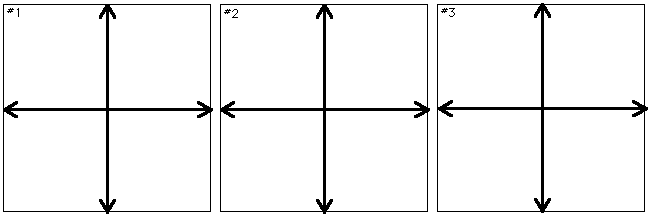Home > General Resources > Recent Talks > Visualizing Change > Solutions to a Differential Equation

# Particular Solutions to a Differential Equation

### Objective

In the previous activity, you developed a slope field corresponding to a differential equation. Slope fields characterize the family of functions that share the rate of change expressed by a differential equation. Each function in this family corresponds to a different constant of integration (a different value of C) in the general algebraic solution to the differential equation. In this activity, you'll explore graphical methods for finding a particular solution to a differential equation. That is, you'll find the specific function (in the family of related functions) that contains a given point.

### Choosing a Differential Equation

If you're exploring these activities in sequential order, you may wish to use the same sketch and the same differential equation for this activity as you used in Activity 5. If so, make sure you follow the steps immediately below. If not, skip these steps and continue reading.

• Reopen the sketch you saved at the end of Activity 5.
• Choose Show All Hidden from the Display menu. Many hidden objects will appear and be selected.
• Hold down the Shift key and very carefully deselect the selected line that passes through Point P. As soon as you've deselected the line (and nothing else), choose Hide Objects from the Display menu to hide the remaining selected objects. This should leave the line visible. If not, close the sketch and repeat these steps.
• Select the line and choose Line Weight: Dashed from the Display menu. You might want to color the line as well. Make sure you're dashing (and coloring) the line passing through P, and not the short collinear segment that also passes through P.
• Turn off the Snap To Grid option on the Graph menu. (If the checkmark is showing next to this menu item, select the item; this will turn off the option.)
• You're ready to proceed. Skip to the "Exploring a Particular Solution" section following the next paragraph.

If you don't have a previous sketch available, or if you otherwise want to start afresh, you should use a pre-made sketch (that already contains a differential equation) in this activity. Find and open the sketch Probe.gsp in the Slopefld folder before continuing. The differential equation used in this sketch is dy/dx = a(x/y), where a is a parameter you can adjust with the slider in the upper corner of the sketch. Choose a new value for a by adjusting the slider, and then proceed with the investigation below.

### Exploring a Particular Solution

Your sketch currently displays a point P and a line passing through it. The line represents the local slope at P determined by a given differential equation. While the differential equation has many (in fact, an infinite number) of solutions, only one of these solutions passes through P. Thus P belongs to a particular solution to the differential equation contained in the sketch.

How can you find the particular solution of which P is a part? You know that the slope line represents the direction of that solution very close to P.

Step 1. With the Selection Arrow tool, drag P a very short distance in the direction that the dashed line is pointing. (As you drag, P leaves behind a "trace" of where it's been.) Notice that as you move along the line, the line begins to change slope. The slope some distance away from your original starting point may be very different from the slope at the starting point. However, if you move slowly and always drag P in the direction that the line is pointing, you can "follow" the changing slope of a particular solution to the differential equation.

Step 2. Try this now. Click and drag P slowly in the direction the dashed slope line is pointing. Do not let go of the mouse, or your trace will disappear! Don't worry about slight mistakes—it's hard to follow the line exactly—but don't let yourself drag too far away from the direction the line is pointing. Keep holding the mouse down while you or your partner reads the next paragraph.

Continue dragging along the (changing) slope line. If you reach the edge of the screen, remember that the slope line actually points in two opposite directions, so now drag back over the trace you've already left and continue in the other direction. Keep going until you either reach the other edge of the screen or until your trace returns to a location near the starting position.

Step 3. You can let go of the mouse now, but be careful not to click anywhere or the trace will disappear. The on-screen trace represents an approximation of the differential equation's particular solution that includes your initial starting point P.

Step 4. Sketch your trace in the first box (#1), below.

Step 5. Now move point P someplace in the sketch away from the current trace and let go. P now represents a point on some other particular solution to the differential equation. Repeat Steps 1–4 to trace the new solution. Then repeat a third time for a third starting place.What do the various solutions to the differential equation have in common? How are they different?